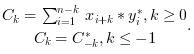Scilab Home page | Wiki | Bug tracker | Forge | Mailing list archives | ATOMS | File exchange
Scilab-Branch-6.1-GIT
Change language to: English - Português - 日本語 - Русский

# xcorr

Computes discrete auto or cross correlation

### Syntax

```[c, lagindex] = xcorr(x)
[c, lagindex] = xcorr(x, y)
[c, lagindex] = xcorr(.., maxlags)
[c, lagindex] = xcorr(.., maxlags, scaling)```

### Parameters

x

a vector of real or complex floating point numbers.

y

a vector of real or complex floating point numbers. The default value is `x`.

maxlags

a scalar with integer value greater than 1. The default value is `n`. Where `n` is the maximum of the `x` and `y` vector length.

scaling

a character string with possible value: `"biased"`, `"unbiased"`, `"coeff"`, `"none"`. The default value is `"none"`.

c

a vector of real or complex floating point numbers with same orientation as `x`.

lagindex

a row vector, containing the lags index corresponding to the `c` values.

### Description

• `c=xcorr(x)` computes the un-normalized discrete auto correlation:and return in `c` the sequence of auto correlation lags Ck=-n:n where `n` is the length of `x`
• `xcorr(x,y)` computes the un-normalized discrete cross correlation:and return in `c` the sequence of auto correlation lags Ck=-n:n where `n` is the maximum of `x` and `y` lengths.

If the `maxlags` argument is given `xcorr` returns in `c` the sequence of auto correlation lags Ck=-maxlags:maxlags. If `maxlags` is greater than `length(x)`, the first and last values of `c` are zero.

The `scaling` argument describes how C(k) is normalized before being returned in `c`:

• "biased": `c=`C`/n`.
• "unbiased": `c=`C`./(n-(-maxlags:maxlags))`.
• "coeff": `c=`C`/(norm(x)*norm(y))`.The corr function computes the "biased" covariance of `x` and `y` and only return in `c` the sequence of auto correlation lags Ck≥0. Method: This function computes C using `ifft(fft(x).*conj(fft(y)))`.

### Examples

```t = linspace(0, 100, 2000);
y = 0.8 * sin(t) + 0.8 * sin(2 * t);
[c, ind] = xcorr(y, "biased");
plot(ind, c)```• xcov — Computes discrete auto or cross covariance
• corr — correlation, covariance
• fft — Transformée de Fourier discrète directe ou inverse uni-, bi-, ou N-dimensionnelle